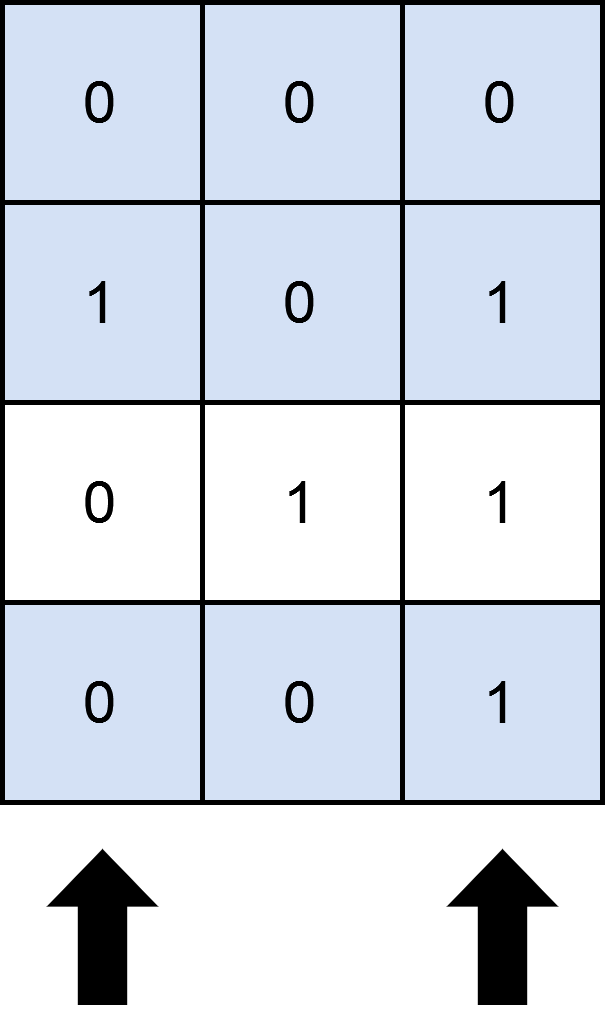##### Welcome to Subscribe On Youtube

Formatted question description: https://leetcode.ca/all/2397.html

# 2397. Maximum Rows Covered by Columns

• Difficulty: Medium.
• Related Topics: Array, Backtracking, Bit Manipulation, Matrix, Enumeration.
• Similar Questions: Matchsticks to Square, Partition to K Equal Sum Subsets, Find the Shortest Superstring, Smallest Sufficient Team, Fair Distribution of Cookies.

## Problem

You are given a 0-indexed m x n binary matrix matrix and an integer numSelect, which denotes the number of distinct columns you must select from matrix.

Let us consider s = {c1, c2, ...., cnumSelect} as the set of columns selected by you. A row row is covered by s if:

• For each cell matrix[row][col] (0 <= col <= n - 1) where matrix[row][col] == 1, col is present in s or,

• No cell in row has a value of 1.

You need to choose numSelect columns such that the number of rows that are covered is maximized.

Return the **maximum number of rows that can be covered by a set of numSelect columns.**

Example 1:Input: matrix = [[0,0,0],[1,0,1],[0,1,1],[0,0,1]], numSelect = 2
Output: 3
Explanation: One possible way to cover 3 rows is shown in the diagram above.
We choose s = {0, 2}.
- Row 0 is covered because it has no occurrences of 1.
- Row 1 is covered because the columns with value 1, i.e. 0 and 2 are present in s.
- Row 2 is not covered because matrix == 1 but 1 is not present in s.
- Row 3 is covered because matrix == 1 and 2 is present in s.
Thus, we can cover three rows.
Note that s = {1, 2} will also cover 3 rows, but it can be shown that no more than three rows can be covered.


Example 2:Input: matrix = [,], numSelect = 1
Output: 2
Explanation: Selecting the only column will result in both rows being covered since the entire matrix is selected.
Therefore, we return 2.


Constraints:

• m == matrix.length

• n == matrix[i].length

• 1 <= m, n <= 12

• matrix[i][j] is either 0 or 1.

• 1 <= numSelect <= n

## Solution (Java, C++, Python)

• class Solution {
public int maximumRows(int[][] mat, int cols) {

int m=mat.length;
int n=mat.length;

int[] s=new int[n];

return f(0,m,n,cols,mat,s);
}

public int f(int ind,int m,int n,int cols,int[][] mat,int[] s){

if(cols==0){
int count=0;
for(int i=0; i<m; i++){
//supposing that this row is valid
boolean selected=true;
for(int j=0; j<n; j++){
//if any cell of this row violates the given two conditions, then the row is discarded
if(mat[i][j]==1 && s[j]!=1)
selected=false;
}
if(selected)
count+=1;
}

return count;
}

int ans=-1;
for(int i=ind; i<n; i++){

s[i]=1; //do
ans=Math.max(ans,f(i+1,m,n,cols-1,mat,s));
s[i]=0; //undo / backtrack
}

return ans;
}
}

############

class Solution {
private int ans;
public int maximumRows(int[][] mat, int cols) {
int m = mat.length, n = mat.length;
int[] arr = new int[m];
for (int i = 0; i < m; ++i) {
int x = 0;
for (int j = 0; j < n; ++j) {
x |= mat[i][j] << j;
}
arr[i] = x;
}
int ans = 0;
continue;
}
int t = 0;
for (int v : arr) {
if ((v & mask) == v) {
++t;
}
}
ans = Math.max(ans, t);
}
return ans;
}
}

• class Solution:
def maximumRows(self, mat: List[List[int]], cols: int) -> int:
arr = []
for i, row in enumerate(mat):
x = 0
for j, v in enumerate(row):
x |= v << j
arr.append(x)
ans, n = 0, len(mat)
for mask in range(1, 1 << n | 1):
continue
t = sum((v & mask) == v for v in arr)
ans = max(ans, t)
return ans

############

# 2397. Maximum Rows Covered by Columns
# https://leetcode.com/problems/maximum-rows-covered-by-columns/

class Solution:
def maximumRows(self, mat: List[List[int]], k: int) -> int:
rows, cols = len(mat), len(mat)
res = 0

A = list(range(cols))

for comb in combinations(A, k):
filled = 0

for row in mat:
ones = row.count(1)
curr = 0

for index in comb:
if row[index] == 1:
curr += 1

if curr == ones:
filled += 1

res = max(res, filled)

return res


• class Solution {
public:
int maximumRows(vector<vector<int>>& mat, int cols) {
int m = mat.size(), n = mat.size();
vector<int> arr(m);
for (int i = 0; i < m; ++i) {
int x = 0;
for (int j = 0; j < n; ++j) x |= mat[i][j] << j;
arr[i] = x;
}
int ans = 0;
int t = 0;
for (int v : arr) t += (v & mask) == v;
ans = max(ans, t);
}
return ans;
}
};

• func maximumRows(mat [][]int, cols int) int {
m, n := len(mat), len(mat)
arr := make([]int, m)
for i, row := range mat {
x := 0
for j, v := range row {
x |= v << j
}
arr[i] = x
}
ans := 0
continue
}
t := 0
for _, v := range arr {
if (v & mask) == v {
t++
}
}
ans = max(ans, t)
}
return ans
}

func max(a, b int) int {
if a > b {
return a
}
return b
}


Explain:

nope.

Complexity:

• Time complexity : O(n).
• Space complexity : O(n).